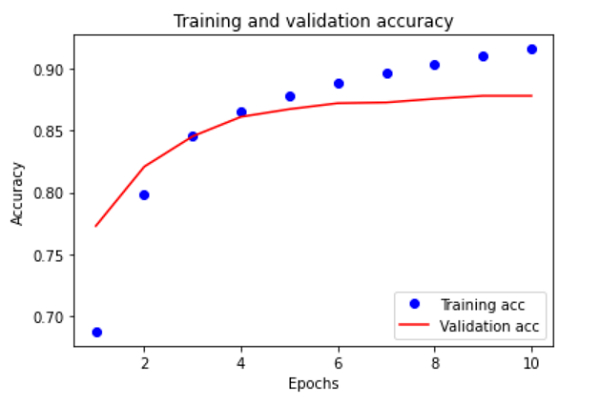# How can TensorFlow be used to create a plot that visualizes training and validation accuracy in the trained IMDB dataset in Python?

PythonServer Side ProgrammingProgramming

Tensorflow is a machine learning framework that is provided by Google. It is an open−source framework used in conjunction with Python to implement algorithms, deep learning applications and much more. It is used in research and for production purposes.

The ‘tensorflow’ package can be installed on Windows using the below line of code −

pip install tensorflow

The ‘IMDB’ dataset contains reviews of over 50 thousand movies. This dataset is generally used with operations associated with Natural Language Processing.

We are using the Google Colaboratory to run the below code. Google Colab or Colaboratory helps run Python code over the browser and requires zero configuration and free access to GPUs (Graphical Processing Units). Colaboratory has been built on top of Jupyter Notebook.

Folloiwng is the code snippet to create a plot that visualizes training and validation accuracy in the trained IMDB dataset −

## Example

plt.plot(epochs, acc, 'bo', label='Training acc')
plt.plot(epochs, val_acc, 'b', label='Validation acc')
plt.title('Training and validation accuracy')
plt.xlabel('Epochs')
plt.ylabel('Accuracy')
plt.legend(loc='lower right')

plt.show()

## Output## Explanation

• Once the data has been fit to the model, the actual values and the predicted values need to be compared.

• The best way to do this is through visualization.

• Hence, the ‘matplotlib’ library is used to plot the accuracy with respect to training and validation datasets.

• This is based on the number of steps (or epochs) taken to train the data to fit the model.